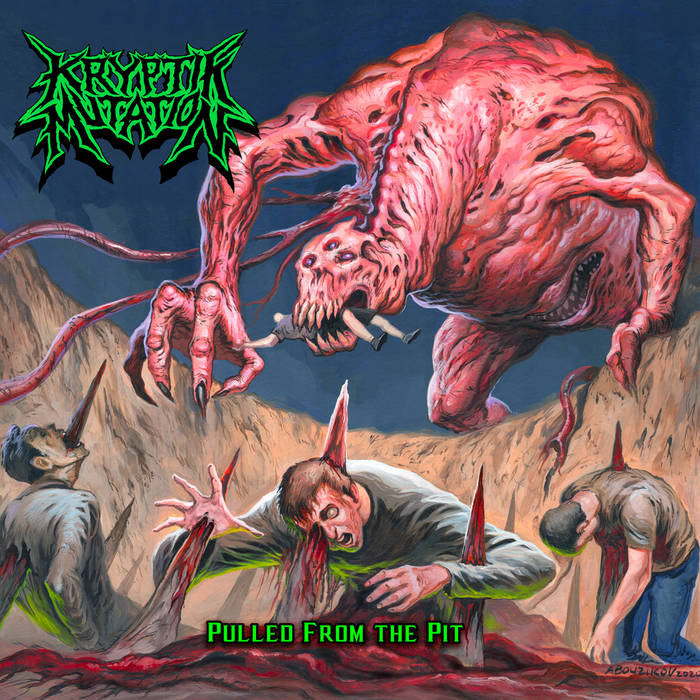## VM-UNDERGROUND

### Extreme Metal Fanzine est. 2012

Filter by: band
[%] - [[0-9]] - [A] - [B] - [C] - [D] - [E] - [F] - [G] - [H] - [I] - [J] - [K] - [L] - [M] - [N] - [O] - [P] - [Q] - [R] - [S] - [T] - [U] - [V] - [W] - [X] - [Y] - [Z]
Filter by: label
[[0-9]] - [A] - [B] - [C] - [D] - [E] - [F] - [G] - [H] - [I] - [J] - [K] - [L] - [M] - [N] - [O] - [P] - [Q] - [R] - [S] - [T] - [U] - [V] - [W] - [X] - [Y] - [Z]
Filter by: style
[B] - [C] - [D] - [E] - [F] - [G] - [H] - [I] - [P] - [S] - [T] - [V]
Filter by: country
[A] - [B] - [C] - [D] - [E] - [F] - [G] - [I] - [L] - [M] - [N] - [P] - [R] - [S] - [T] - [U]
Filter by: vmu-author
[A] - [B] - [C] - [D] - [E] - [F] - [G] - [H] - [I] - [J] - [K] - [L] - [M] - [N] - [O] - [P] - [R] - [S] - [T] - [V] - [X] - [Y] - [Z]

# Kryptik Mutation – Pulled from the Pit## Info

2019 was the year that Death Metal got a boost in McAllen, Texas (USA), the hometown of Severance, one of the known acts of the TXDM movement, as Kryptik Mutation got formed.

On “Pulled from the Pit” you will hear a combination of the 90s US Death Metal scene, along with the then Thrashin’ hybrid as well, with the more modern Brutal Death Metal style to create a groovy mutated beast.

So if Suffocation, Malevolent Creation and Devastation sounds good to you, take a listen to “Pulled from the Pit” to add another –tion bandname to your collection. (Ricardo)